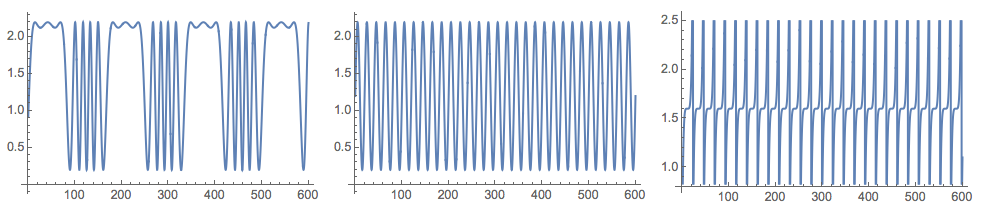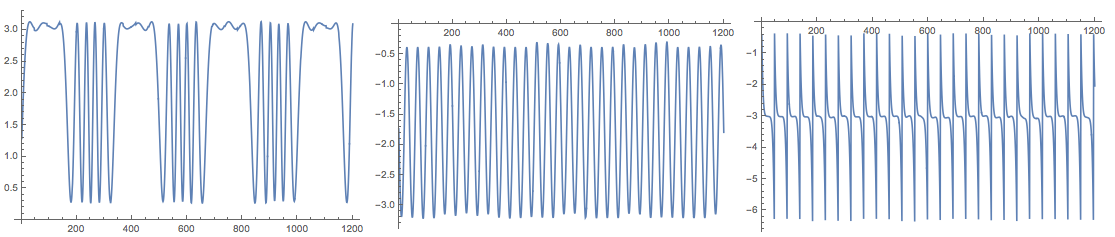# Independent component analysis for multidimensional signals

## Introduction

Independent Component Analysis (ICA) is a (matrix factorization) method for separation of a multi-dimensional signal (represented with a matrix) into a weighted sum of sub-components that have less entropy than the original variables of the signal. See [1,2] for introduction to ICA and more details.

This blog post is to proclaim the implementation of the "FastICA" algorithm in the package IndependentComponentAnalysis.m and show a basic application with it. (I programmed that package last weekend. It has been in my ToDo list to start ICA algorithms implementations for several months… An interesting offshoot was the procedure I derived for the StackExchange question "Extracting signal from Gaussian noise".)

In this blog post ICA is going to be demonstrated with both generated data and "real life" weather data (temperatures of three cities within one month).

## Generated data

In order to demonstrate ICA let us make up some data in the spirit of the "cocktail party problem".

``````(*Signal functions*)
Clear[s1, s2, s3]
s1[t_] := Sin[600 \[Pi] t/10000 + 6*Cos[120 \[Pi] t/10000]] + 1.2
s2[t_] := Sin[\[Pi] t/10] + 1.2
s3[t_?NumericQ] := (((QuotientRemainder[t, 23][] - 11)/9)^5 + 2.8)/2 + 0.2

(*Mixing matrix*)
A = {{0.44, 0.2, 0.31}, {0.45, 0.8, 0.23}, {0.12, 0.32, 0.71}};

(*Signals matrix*)
nSize = 600;
S = Table[{s1[t], s2[t], s3[t]}, {t, 0, nSize, 0.5}];

(*Mixed signals matrix*)
M = A.Transpose[S];

(*Signals*)
Grid[{Map[
Plot[#, {t, 0, nSize}, PerformanceGoal -> "Quality",
ImageSize -> 250] &, {s1[t], s2[t], s3[t]}]}]````````````(*Mixed signals*)
Grid[{Map[ListLinePlot[#, ImageSize -> 250] &, M]}]``````I took the data generation formulas from .

## ICA application

``Import["https://raw.githubusercontent.com/antononcube/MathematicaForPrediction/master/IndependentComponentAnalysis.m"]``

It is important to note that the usual ICA model interpretation for the factorized matrix X is that each column is a variable (audio signal) and each row is an observation (recordings of the microphones at a given time). The matrix 3×1201 M was constructed with the interpretation that each row is a signal, hence we have to transpose M in order to apply the ICA algorithms, X=M^T.

``````X = Transpose[M];

{S, A} = IndependentComponentAnalysis[X, 3];``````

Check the approximation of the obtained factorization:

``````Norm[X - S.A]
(* 3.10715*10^-14 *)``````

Plot the found source signals:

``````Grid[{Map[ListLinePlot[#, PlotRange -> All, ImageSize -> 250] &,
Transpose[S]]}]``````Because of the random initialization of the inverting matrix in the algorithm the result my vary. Here is the plot from another run:The package also provides the function `FastICA` that returns an association with elements that correspond to the result of the function `fastICA` provided by the R package "fastICA". See .

Here is an example usage:

``````res = FastICA[X, 3];

Keys[res]
(* {"X", "K", "W", "A", "S"} *)

Grid[{Map[
ListLinePlot[#, PlotRange -> All, ImageSize -> Medium] &,
Transpose[res["S"]]]}]``````Note that (in adherence to ) the function `FastICA` returns the matrices S and A for the centralized matrix X. This means, for example, that in order to check the approximation proper mean has to be supplied:

``````Norm[X - Map[# + Mean[X] &, res["S"].res["A"]]]
(* 2.56719*10^-14 *)``````

## Signatures and results

The result of the function `IndependentComponentAnalysis` is a list of two matrices. The result of `FastICA` is an association of the matrices obtained by ICA. The function `IndependentComponentAnalysis` takes a method option and options for precision goal and maximum number of steps:

``````In:= Options[IndependentComponentAnalysis]

Out= {Method -> "FastICA", MaxSteps -> 200, PrecisionGoal -> 6}``````

The intent is `IndependentComponentAnalysis` to be the front interface to different ICA algorithms. (Hence, it has a Method option.) The function `FastICA` takes as options the named arguments of the R function `fastICA` described in .

``````In:= Options[FastICA]

Out= {"NonGaussianityFunction" -> Automatic,
"NegEntropyFactor" -> 1, "InitialUnmixingMartix" -> Automatic,
"RowNorm" -> False, MaxSteps -> 200, PrecisionGoal -> 6,
"RFastICAResult" -> True}``````

At this point `FastICA` has only the deflation algorithm described in . ( has also the so called "symmetric" ICA sub-algorithm.) The R function `fastICA` in  can use only two neg-Entropy functions log(cosh(x)) and exp(-u^2/2). Because of the symbolic capabilities of Mathematica `FastICA` of  can take any listable function through the option "NonGaussianityFunction", and it will find and use the corresponding first and second derivatives.

## Using NNMF for ICA

It seems that in some cases, like the generated data used in this blog post, Non-Negative Matrix Factorization (NNMF) can be applied for doing ICA.

To be clear, NNMF does dimension reduction, but its norm minimization process does not enforce variable independence. (It enforces non-negativity.) There are at least several articles discussing modification of NNMF to do ICA. For example .

Load NNMF package  (from MathematicaForPrediction at GitHub):

``Import["https://raw.githubusercontent.com/antononcube/MathematicaForPrediction/master/NonNegativeMatrixFactorization.m"]``

After several applications of NNMF we get signals close to the originals:

``````{W, H} = GDCLS[M, 3];
Grid[{Map[ListLinePlot[#, ImageSize -> 250] &, Normal[H]]}]``````For the generated data in this blog post, FactICA is much faster than NNMF and produces better separation of the signals with every run. The data though is a typical representative for the problems ICA is made for. Another comparison with image de-noising, extending my previous blog post, will be published shortly.

## ICA for mixed time series of city temperatures

Using Mathematica‘s function `WeatherData` we can get temperature time series for a small set of cities over a certain time grid. We can mix those time series into a multi-dimensional signal, MS, apply ICA to MS, and judge the extracted source signals with the original ones.

This is done with the following commands.

### Get time series data

``````cities = {"Sofia", "London", "Copenhagen"};
timeInterval = {{2016, 1, 1}, {2016, 1, 31}};
ts = WeatherData[#, "Temperature", timeInterval] & /@ cities;

opts = {PlotTheme -> "Detailed", FrameLabel -> {None, "temperature,\[Degree]C"}, ImageSize -> 350};
DateListPlot[ts,
PlotLabel -> "City temperatures\nfrom " <> DateString[timeInterval[], {"Year", ".", "Month", ".", "Day"}] <>
" to " <> DateString[timeInterval[], {"Year", ".", "Month", ".", "Day"}],
PlotLegends -> cities, ImageSize -> Large, opts]``````### Cleaning and resampling (if any)

Here we check the data for missing data:

``````Length /@ Through[ts["Path"]]
Count[#, _Missing, \[Infinity]] & /@ Through[ts["Path"]]
Total[%]
(* {1483, 1465, 742} *)
(* {0,0,0} *)
(* 0 *)``````

Resampling per hour:

``ts = TimeSeriesResample[#, "Hour", ResamplingMethod -> {"Interpolation", InterpolationOrder -> 1}] & /@ ts``

### Mixing the time series

In order to do a good mixing we select a mixing matrix for which all column sums are close to one:

``````mixingMat = #/Total[#] & /@ RandomReal[1, {3, 3}];
MatrixForm[mixingMat]
(* mixingMat = {{0.357412, 0.403913, 0.238675}, {0.361481, 0.223506, 0.415013}, {0.36564, 0.278565, 0.355795}} *)
Total[mixingMat]
(* {1.08453, 0.905984, 1.00948} *)``````

Note the row normalization.

Make the mixed signals:

``tsMixed = Table[TimeSeriesThread[mixingMat[[i]].# &, ts], {i, 3}]``

Plot the original and mixed signals:

``````Grid[{{DateListPlot[ts, PlotLegends -> cities, PlotLabel -> "Original signals", opts],
DateListPlot[tsMixed, PlotLegends -> Automatic, PlotLabel -> "Mixed signals", opts]}}]
``````### Application of ICA

At this point we apply ICA (probably more than once, but not too many times) and plot the found source signals:

``````X = Transpose[Through[tsMixed["Path"]][[All, All, 2]] /. Quantity[v_, _] :> v];
{S, A} = IndependentComponentAnalysis[X, 3];
DateListPlot[Transpose[{tsMixed[]["Dates"], #}], PlotTheme -> "Detailed", ImageSize -> 250] & /@ Transpose[S]``````Compare with the original time series:

``MapThread[DateListPlot[#1, PlotTheme -> "Detailed", PlotLabel -> #2, ImageSize -> 250] &, {tsPaths, cities}]``After permuting and inverting some of the found sources signals we see they are fairly good:

``````pinds = {3, 1, 2};
pmat = IdentityMatrix[[All, pinds]];

DateListPlot[Transpose[{tsMixed[]["Dates"], #}], PlotTheme -> "Detailed", ImageSize -> 250] & /@
Transpose[S.DiagonalMatrix[{1, -1, 1}].pmat]``````A. Hyvarinen and E. Oja (2000) Independent Component Analysis: Algorithms and Applications, Neural Networks, 13(4-5):411-430 . URL: https://www.cs.helsinki.fi/u/ahyvarin/papers/NN00new.pdf .

 Wikipedia entry, Independent component analysis, URL: https://en.wikipedia.org/wiki/Independent_component_analysis .

 A. Antonov, Independent Component Analysis Mathematica package, (2016), source code MathematicaForPrediction at GitHub, package IndependentComponentAnalysis.m .

 J. L. Marchini, C. Heaton and B. D. Ripley, fastICA, R package, URLs: https://cran.r-project.org/web/packages/fastICA/index.html, https://cran.r-project.org/web/packages/fastICA/fastICA.pdf .

 A. Antonov, Implementation of the Non-Negative Matrix Factorization algorithm in Mathematica, (2013), source code at MathematicaForPrediction at GitHub, package NonNegativeMatrixFactorization.m.

 H. Hsieh and J. Chien, A new nonnegative matrix factorization for independent component analysis, (2010), Conference: Acoustics Speech and Signal Processing (ICASSP).

## 15 thoughts on “Independent component analysis for multidimensional signals”

1.Markus Röllig says:

Hey, thanks for providing the package. I tried to apply it to a set of mixed sound files from this site: https://research.ics.aalto.fi/ica/cocktail/cocktail_en.cgi
However, I can not replicate the demixed signals. Can you give me a hint or two how to improve the results?

•Anton Antonov Antonov says:

Thanks!

Were you able to reproduce the computations given in the blog post? How about the ICA computations given in these Mathematica StackExchage posts:

“How to do Independent Component Analysis?”

“How to perform Blind Source Separation on an audio mix?”

Using the code in the latter link I was able to successfully retrieve one of the signals (of Dr Jarmo Hurri) of mixtures with names “111000000mix*.wav”.(I.e. mixtures of a cop car siren, CNN news man, and Dr Jarmo Hurri). The other two — the cop car siren and the CNN news caster — were too entangled. It is probably possible to apply ICA for a second time only on the corresponding two ICA result columns in order to separate them.

•Markus Röllig says:

Wow, quick reply :). Yes I am able to reproduce the results from your examples.

However, when I do some experiments by my own the results are less convincing. Take for example three signals ( I omit the noise here):

f1 = Sin;
f2 = (2 Mod[#/10, 1] – 1) &;
f3 = (-1/2 Sign[Sin[[Pi] #/4]]) &;
z1 = f1 /@ Range[0, 20, 0.01];
z2 = f2 /@ Range[0, 20, 0.01];
z3 = f3 /@ Range[0, 20, 0.01];
z = Transpose[{z1, z2, z3}];
xT = a.Transpose[z];
fi = FastICA[Transpose@xT, 3];

Then one of the three unmixed signals is usually very well reproduced, while the other two are still significantly mixed.

ListPlot[Transpose[fi[“S”]][[#]], Joined -> True]&/@{1,2,3}

This is even more pronounced when I download the mixed party sounds from the website above. I can not get rid of the police siren for example, which is hardly noticeable in their demixed signal.

•Anton Antonov Antonov says:

•Markus Röllig says:

Right. That is why I was asking in the first place. They provide demixed signals of a quality that i can not reproduce – even for a single file. They remain contaminated. I was hoping to reproduce it with some arcane Option values 🙂

•Anton Antonov Antonov says:

Please see this ICA run with your second example. I had to define the matrix `a`. Also, I used higher precision goal. So, I think you might have get better results by re-mixing the mixed signals, changing the values of the precision goal, using different random seeds.

2.Markus Röllig says:

Are you aware of the underlying reason for not being able to demix the three artificial signals I gave? Similar examples from some of the publications from the Helsinki group seem to be able to apply ICA on similar data with much better results.

3.Markus Röllig says:

Thank you. I seems a little random, because several consecutive runs fail to come up with the same results. I am surprised that the random initial matrix guess is of such a strong impact.
I will continue playing with it – thanks!

4.Aliakbar A. says:

What is the principle (in a mathematical sense, e.g. convolution or something else …) behind mixing the three Signals?

•Anton Antonov Antonov says:

In a mathematical sense if you have a set of microphones, then each microphone has its own weighted sum of the signals it has picked. With ICA we try to identify a vector basis using a set of (different) weighted sums of basis` elements.

5.EigenHorizons (@EigenHorizons) says:

How do we generate the Mixing matrix ? what is the rationale behind it?

•Anton Antonov Antonov says:

The mixing matrix in the example was hand-made in order to provide a good illustration. The rationale comes from the blind source separation problem.

•EigenHorizons (@EigenHorizons) says:

Thank you so much!

This site uses Akismet to reduce spam. Learn how your comment data is processed.